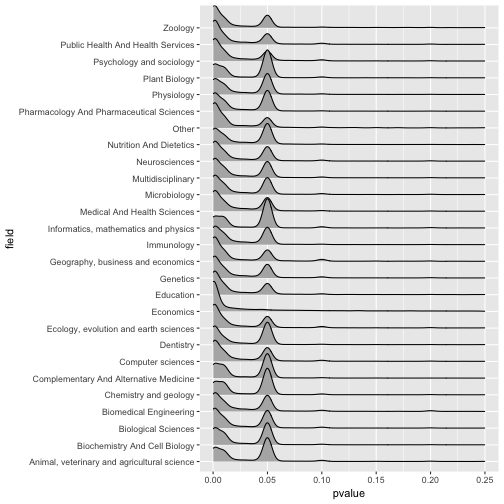1979年，英国乐队快乐小分队（Joy Division）发行了自己的首张唱片《Unknown Pleasuers》，这张专辑发行两周内就卖了5000份，但问题是……印了10000份。然而，当乐队的单曲《Transmission》发布后，这张后朋克唱片很快销售一空。作为一个乐盲，我是没搞懂这歌的意思（好像对收音机很不满）。整个70年代不断衰落的英国社会使青少年群体对现实极度不满，采取了一些很强烈的表现形式来抒发感情，这催生了朋克运动，至于后朋克运动，据说比朋克更具实验性质。有意思的是这个专辑在2017年又重新流行了，倒不是因为社会再度衰落，而是那个设计极为特殊的封面。《Unknown Pleasuers》封面（图片来自维基百科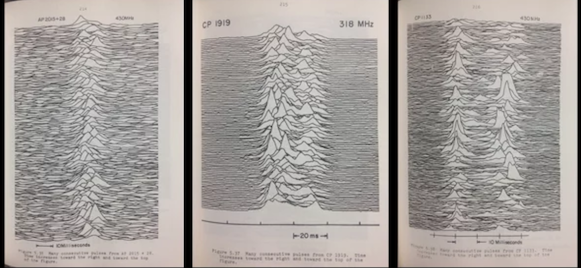《Unknown Pleasuers》封面的源头，Harold D. Craft, Jr. 博士论文插图。Radio Observations of the Pulse Profiles and Dispersion Measures of Twelve Pulsars, Harold D. Craft, Jr. (PhD Thesis, September 1970 pages 214-216), Cornell University

it’s my image, and I ought to have a copy of it.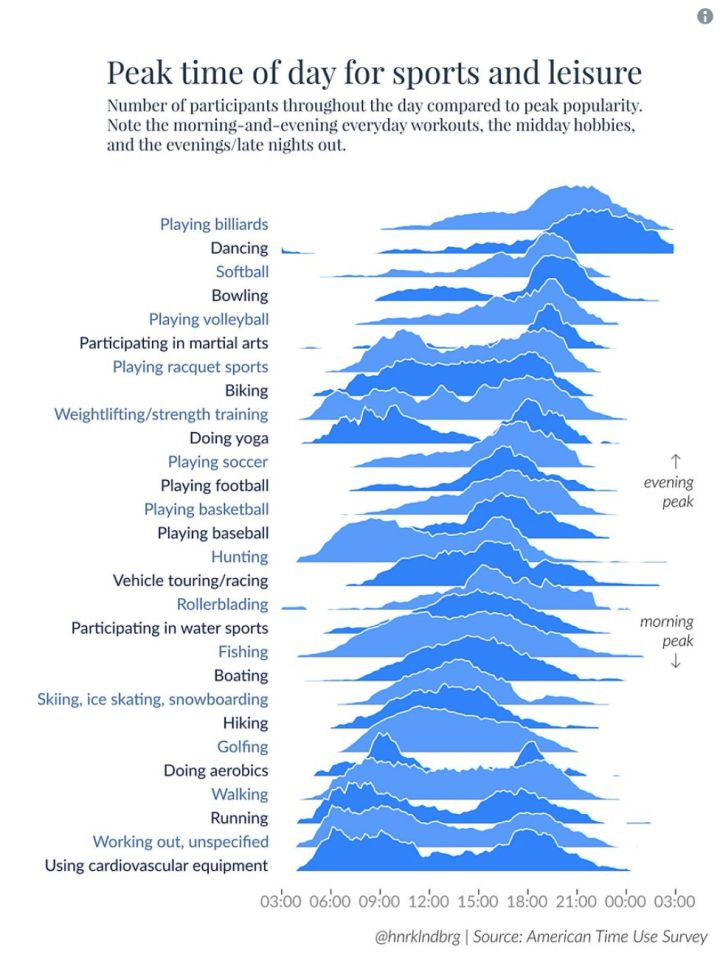group1 <- cbind(rnorm(1000), 1)
group2 <- cbind(runif(1000, min = -1, max = 1), 2)
group3 <- cbind(c(rnorm(500, 1, 0.5),rnorm(500, -1, 0.5)), 3)
data <- rbind.data.frame(group1, group2, group3)


library(tidyverse)
data %>%
group_by(V2) %>%
summarise(mean = mean(V1)) %>%
ungroup() %>%
ggplot(aes(x = V2, y = mean)) +
geom_bar(stat = 'identity')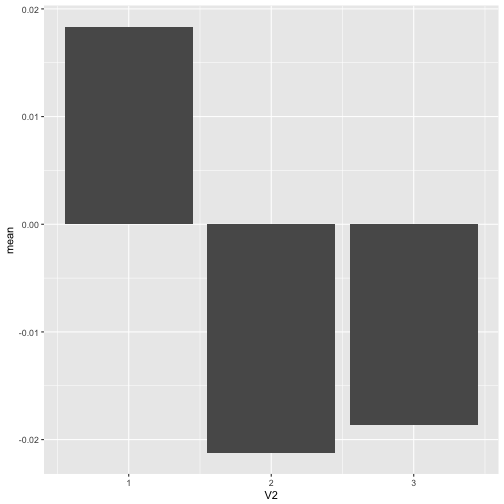data %>%
ggplot(aes(x = factor(V2), y = V1)) +
geom_boxplot()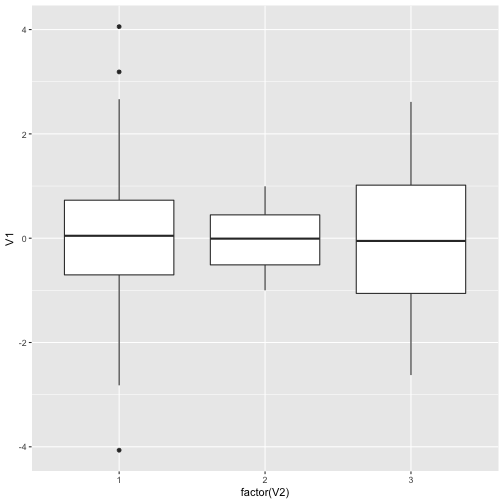data %>%
ggplot(aes(x = factor(V2), y = V1)) +
geom_violin()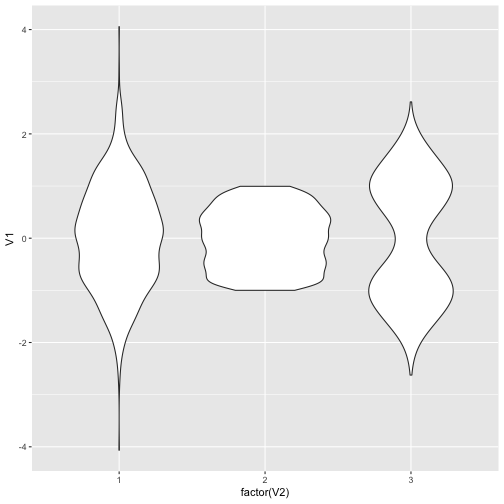data %>%
ggplot(aes(x = factor(V2), y = V1)) +
geom_jitter()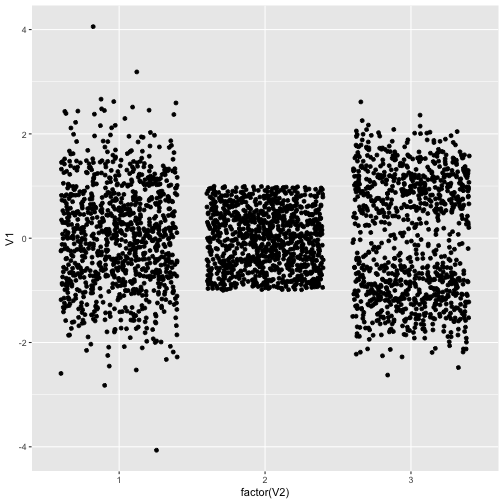library(ggridges)
data %>%
ggplot(aes(y = factor(V2), x = V1)) +
geom_density_ridges()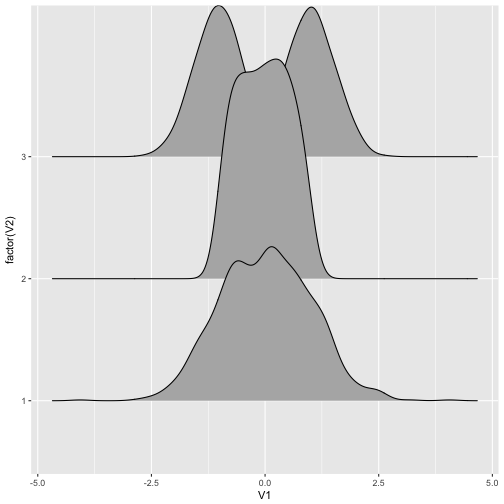data %>%
ggplot(aes(y = factor(V2), x = V1),height = ..density..) +
geom_density_ridges(stat = 'binline')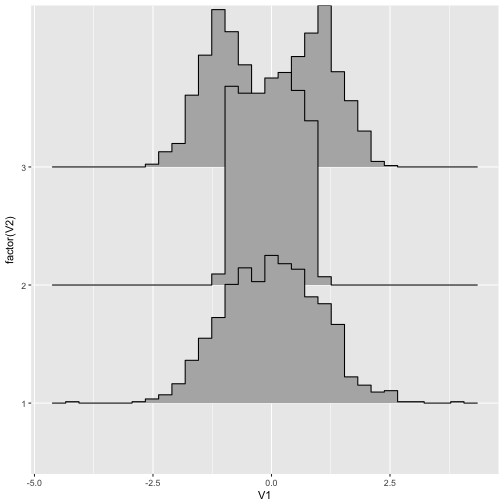library(tidypvals)
aj1 = anti_join(head2015, chavalarias2016)
aj2 = anti_join(chavalarias2016, head2015)
sj1 = semi_join(head2015, chavalarias2016)
allp = rbind(aj1, aj2, sj1)

allp = rbind(allp, brodeur2016)

allp %>%
filter(!is.na(field)) %>%
ggplot(aes(y = field, x = pvalue)) +
geom_density_ridges() + xlim(0, 0.25)